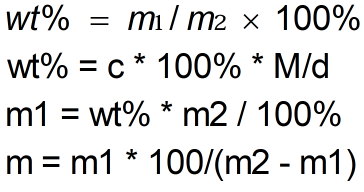Request a Tool

Concentration Calculator

The amount of solute in a solution that is relative to the total amount of solute in the solution

Output

Percentage concentration
0
Mass per 100 g H2O
0

Formula• D = Density
• c = Molarity
• M = Molar Mass
• mass of solution m₂ = 100
• m1 = mass of solute

Defination / Uses

The concentration of a solution is the ratio of the solute to the solvent or total solution. Concentration is a term that describes the composition of a solution, most frequently a water solution, but may also be used to describe the mixture of any composition.

How to Calculate Percentage Concentration ?

1. Enter the amount of Density
2. Enter the amount Molarity
3. Enter the amount Molar Mass
4. In Result displays the Percentage concentration and Mass per 100 g H2O

Use the upper given formula for manual calculations. No sign-up, registration OR captcha is required to use this tool.